## The Calculator Encyclopedia now has a Return On Investment (ROI) Calculator

First and Foremost, what is a Return On Investment?

According to Investopedia,

Return on Investment (ROI) is a performance measure used to evaluate the efficiency of an investment or compare the efficiency of a number of different investments. ROI tries to directly measure the amount of return on an particular investment, relative to the investment’s cost. To calculate ROI, the benefit (or return) of an investment is divided by the cost of the investment. The result is expressed as a percentage or a ratio.

According to Wikipedia,

Return on investment (ROI) is a ratio between the net profit and cost of investment resulting from an investment of some resources. A high ROI means the investment’s gains favorably to its cost. As a performance measure, ROI is used to evaluate the efficiency of an investment or to compare the efficiencies of several different investments. In purely economic terms, it is one way of relating profits to capital invested. Return on investment is a performance measure used by businesses to identify the efficiency of an investment or number of different investments.

Return on investment (ROI) measures the gain or loss generated on an investment relative to the amount of money invested. ROI is usually expressed as a percentage and is typically used for personal financial decisions, to compare a company’s profitability or to compare the efficiency of different investments.

Nickzom Calculator+ (Professional Version) – The Calculator Encyclopedia solves ROI calculations and gives one the annualized ROI.

Like every other calculations there are parameters you need to input its values to get a certain result.

The parameters for Nickzom Calculator+ ROI (Return On Investment) are:

• Amount Invested
• Amount Returned
• Investment Length (Number of Years)

One can access Nickzom Calculator+ (Professional Version) – The Calculator Encyclopedia via any of these channels:

Let’s get started,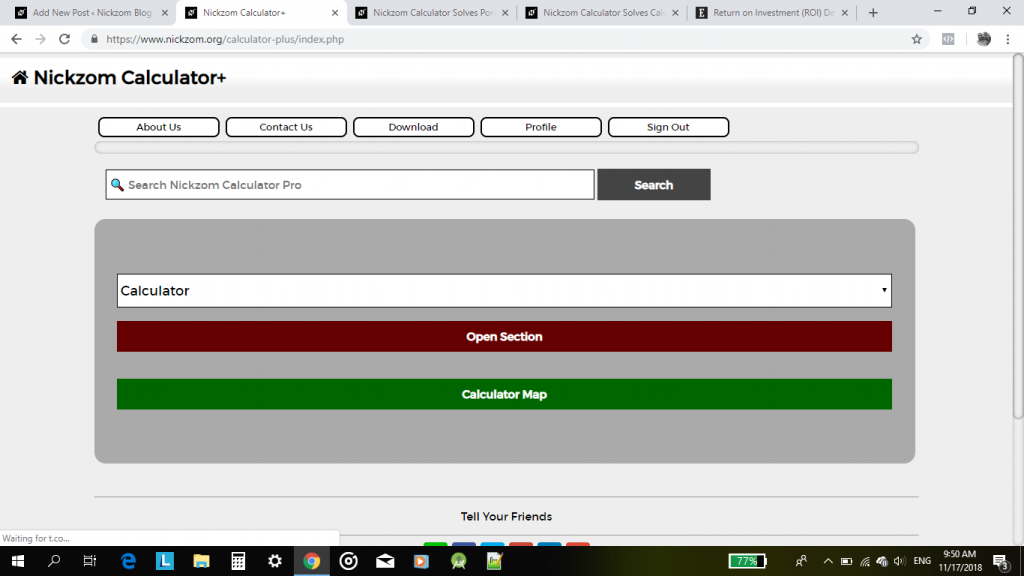First, Click on Calculator Map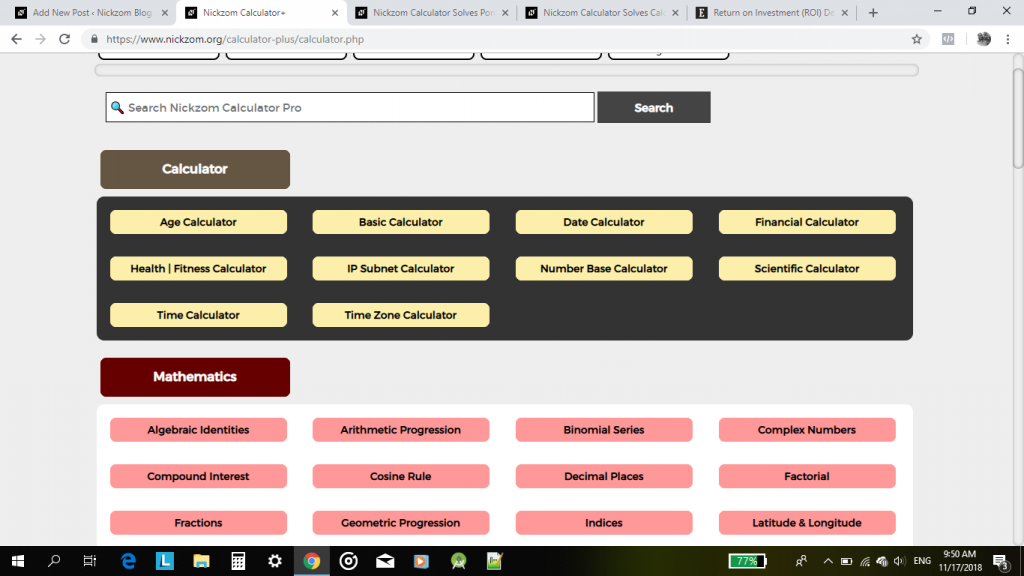Now, click on Financial Calculator,Click on ROI CalculatorNow, enter the values of the parameters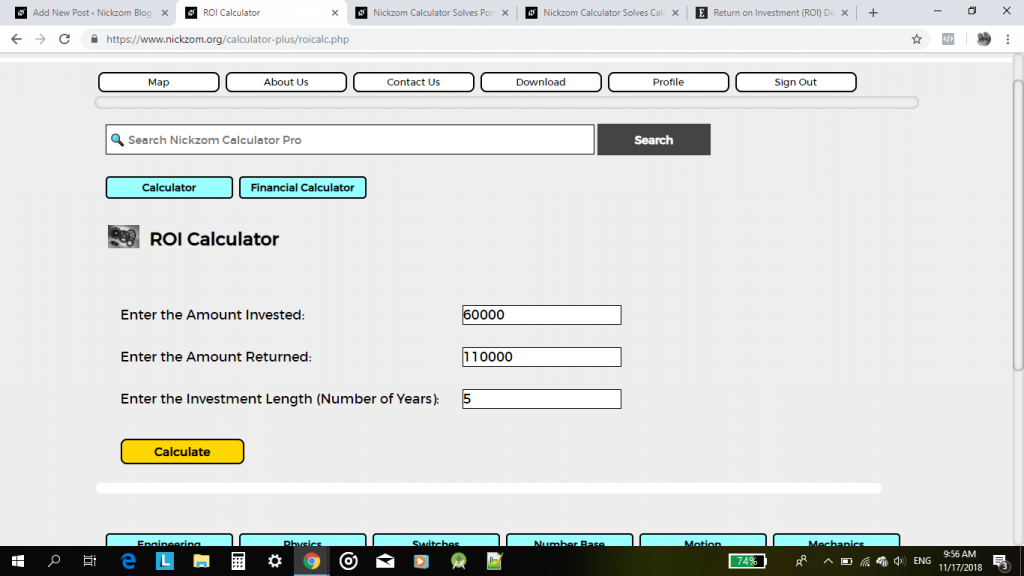Click on Calculate button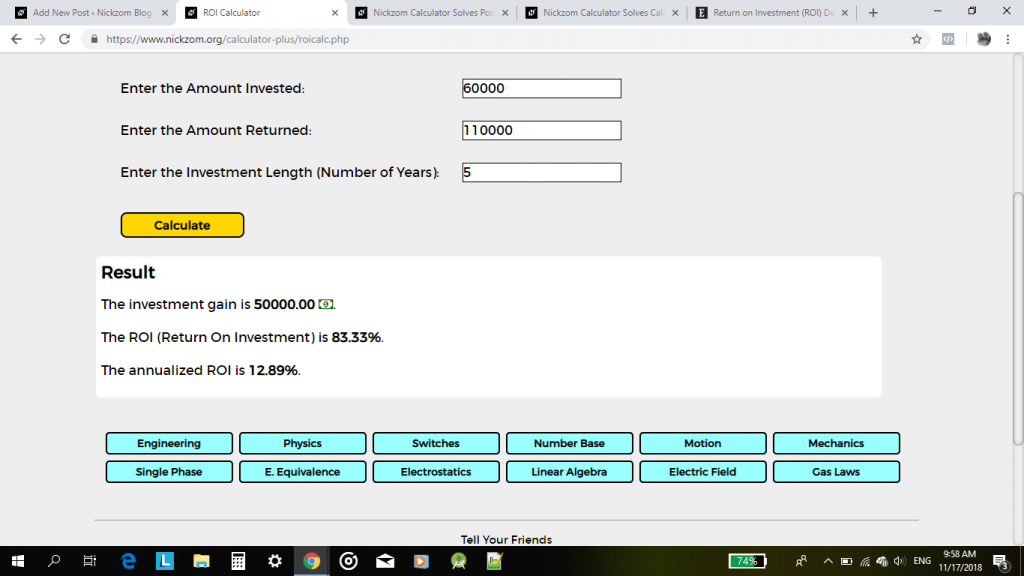There you have it, very simple and easy going with an accurate answer.

## Nickzom Calculator Solves Porosity in Sonic Log under Geology – The Calculator Encyclopedia

According to Wikipedia,

Sonic logging is a well logging tool that provides a formation’s interval transit time, designated as, which is a measure of a formation’s capacity to transmit seismic waves. Geologically, this capacity varies with lithology and rock textures, most notably decreasing with an increasing effective porosity

Nickzom Calculator+ (Professional Version) – The Calculator Encyclopedia solves calculations on porosity for sonic log and shows the steps carefully.

The parameters to input to get the answer for your porosity problem are:

• Internal Transit Time of Formation
• Internal Transit Time of Matrix
• Internal Transit Time of the Fluid in the Well Bore
• Compaction Factor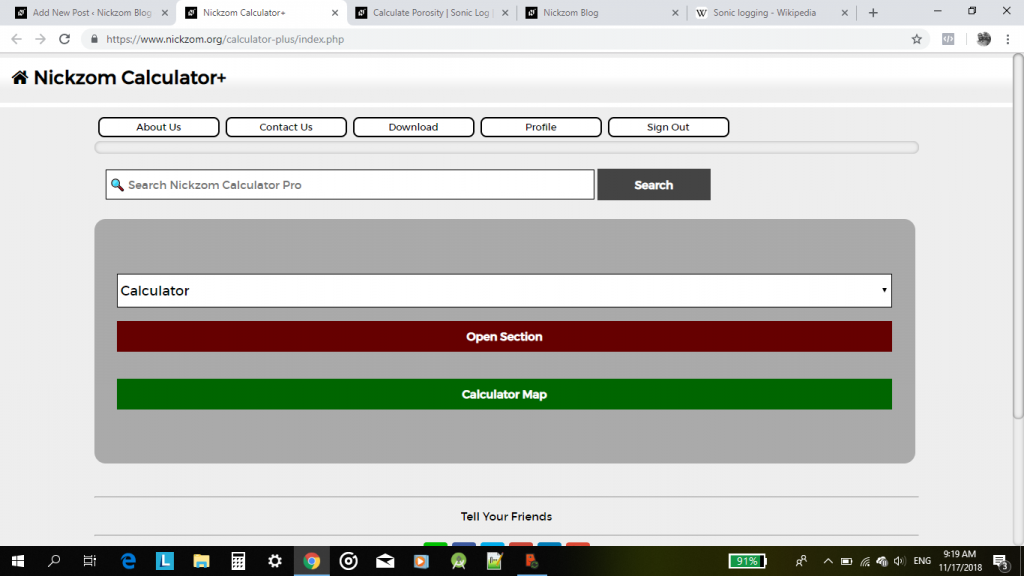Select Geology from the drop down.